# Mercury Receiver Implementation in Verilog Kirk Weedman KD

• Slides: 22Mercury Receiver Implementation in Verilog Kirk Weedman KD 7 IRSStages: 5 Decimation: 80/160/320 VARCIC Stages: 11 Decimation: 4 Decimation: 2 CIC FIR rate 2 CORDIC 1 Out_strobe In_strobe I I ADC Data 22 Q 23 Frequency 122. 88 MHz clock 24 FIR Q 23 Out_strobe 1 CIC VARCIC 22 32 24 In_strobe 24 24 In_strobe Out_strobe In_strobe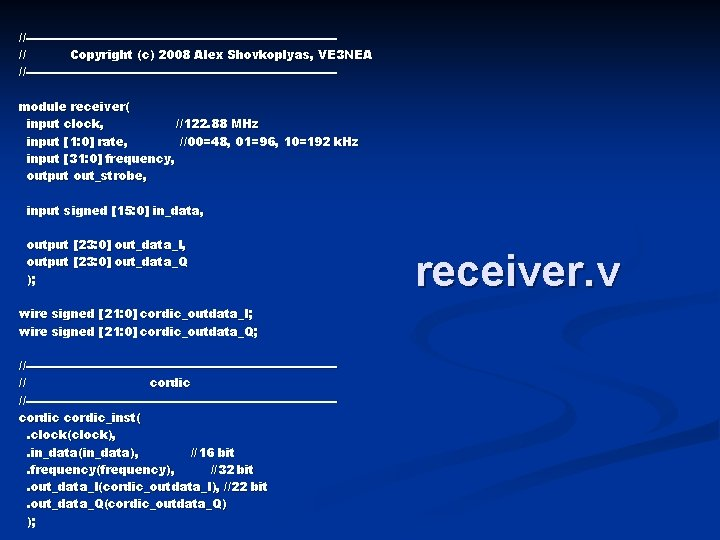//---------------------------------------// Copyright (c) 2008 Alex Shovkoplyas, VE 3 NEA //---------------------------------------module receiver( input clock, //122. 88 MHz input [1: 0] rate, //00=48, 01=96, 10=192 k. Hz input [31: 0] frequency, output out_strobe, input signed [15: 0] in_data, output [23: 0] out_data_I, output [23: 0] out_data_Q ); wire signed [21: 0] cordic_outdata_I; wire signed [21: 0] cordic_outdata_Q; //---------------------------------------// cordic //---------------------------------------cordic_inst(. clock(clock), . in_data(in_data), //16 bit. frequency(frequency), //32 bit. out_data_I(cordic_outdata_I), //22 bit. out_data_Q(cordic_outdata_Q) ); receiver. v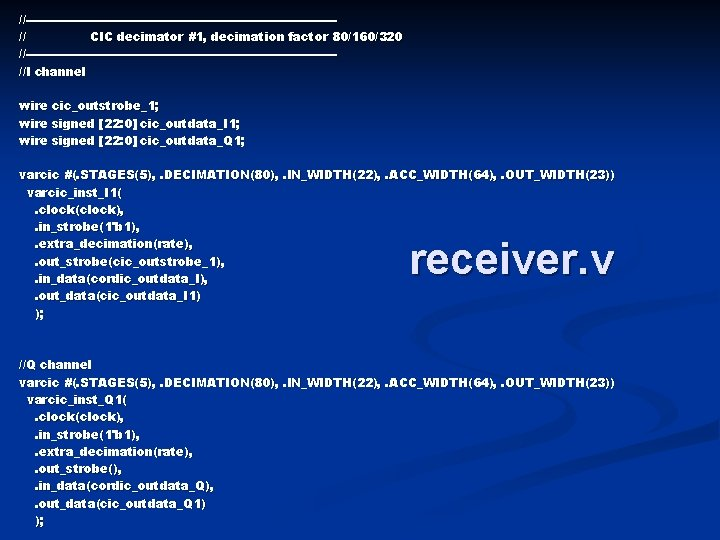//---------------------------------------// CIC decimator #1, decimation factor 80/160/320 //---------------------------------------//I channel wire cic_outstrobe_1; wire signed [22: 0] cic_outdata_I 1; wire signed [22: 0] cic_outdata_Q 1; varcic #(. STAGES(5), . DECIMATION(80), . IN_WIDTH(22), . ACC_WIDTH(64), . OUT_WIDTH(23)) varcic_inst_I 1(. clock(clock), . in_strobe(1'b 1), . extra_decimation(rate), . out_strobe(cic_outstrobe_1), . in_data(cordic_outdata_I), . out_data(cic_outdata_I 1) ); receiver. v //Q channel varcic #(. STAGES(5), . DECIMATION(80), . IN_WIDTH(22), . ACC_WIDTH(64), . OUT_WIDTH(23)) varcic_inst_Q 1(. clock(clock), . in_strobe(1'b 1), . extra_decimation(rate), . out_strobe(), . in_data(cordic_outdata_Q), . out_data(cic_outdata_Q 1) );//---------------------------------------// CIC decimator #2, decimation factor 4 //---------------------------------------//I channel wire cic_outstrobe_2; wire signed [23: 0] cic_outdata_I 2; wire signed [23: 0] cic_outdata_Q 2; cic #(. STAGES(11), . DECIMATION(4), . IN_WIDTH(23), . ACC_WIDTH(45), . OUT_WIDTH(24)) cic_inst_I 2(. clock(clock), . in_strobe(cic_outstrobe_1), . out_strobe(cic_outstrobe_2), . in_data(cic_outdata_I 1), . out_data(cic_outdata_I 2) ); receiver. v //Q channel cic #(. STAGES(11), . DECIMATION(4), . IN_WIDTH(23), . ACC_WIDTH(45), . OUT_WIDTH(24)) cic_inst_Q 2(. clock(clock), . in_strobe(cic_outstrobe_1), . out_strobe(), . in_data(cic_outdata_Q 1), . out_data(cic_outdata_Q 2) );//---------------------------------------// FIR coefficients and sequencing //---------------------------------------wire signed [23: 0] fir_coeff; fir_coeffs_inst(. clock(clock), . start(cic_outstrobe_2), . coeff(fir_coeff) ); //---------------------------------------// FIR decimator //---------------------------------------fir #(. OUT_WIDTH(24)) fir_inst_I(. clock(clock), . start(cic_outstrobe_2), . coeff(fir_coeff), . in_data(cic_outdata_I 2), . out_data(out_data_I), . out_strobe(out_strobe) ); fir #(. OUT_WIDTH(24)) fir_inst_Q(. clock(clock), . start(cic_outstrobe_2), . coeff(fir_coeff), . in_data(cic_outdata_Q 2), . out_data(out_data_Q), . out_strobe() ); endmodule receiver. vStages: 5 Decimation: 80/160/320 VARCIC Stages: 11 Decimation: 4 Decimation: 2 CIC FIR rate 2 CORDIC 1 Out_strobe In_strobe I I ADC Data 22 Q 23 Frequency 122. 88 MHz clock 24 FIR Q 23 Out_strobe 1 CIC VARCIC 22 32 24 In_strobe 24 24 In_strobe Out_strobe In_strobeCIC Filters A CIC decimator has N cascaded integrator stages clocked at fs, followed by a rate change factor of R, followed by N cascaded comb stages running at fs/R I I I R C Three Stage Decimating CIC Filter (N = 3) C C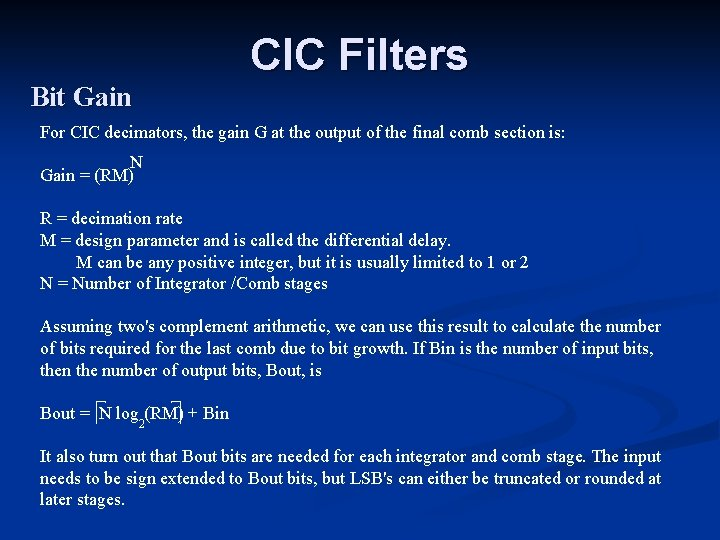CIC Filters Bit Gain For CIC decimators, the gain G at the output of the final comb section is: N Gain = (RM) R = decimation rate M = design parameter and is called the differential delay. M can be any positive integer, but it is usually limited to 1 or 2 N = Number of Integrator /Comb stages Assuming two's complement arithmetic, we can use this result to calculate the number of bits required for the last comb due to bit growth. If Bin is the number of input bits, then the number of output bits, Bout, is Bout = N log (RM) + Bin 2 It also turn out that Bout bits are needed for each integrator and comb stage. The input needs to be sign extended to Bout bits, but LSB's can either be truncated or rounded at later stages.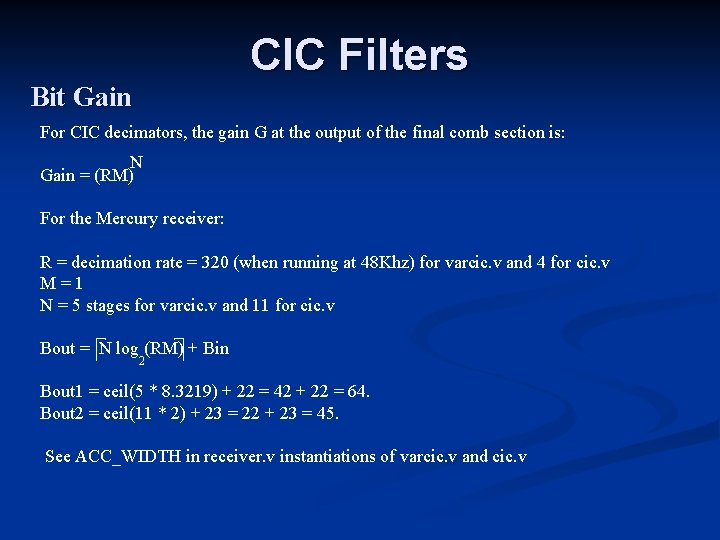CIC Filters Bit Gain For CIC decimators, the gain G at the output of the final comb section is: N Gain = (RM) For the Mercury receiver: R = decimation rate = 320 (when running at 48 Khz) for varcic. v and 4 for cic. v M=1 N = 5 stages for varcic. v and 11 for cic. v Bout = N log (RM) + Bin 2 Bout 1 = ceil(5 * 8. 3219) + 22 = 42 + 22 = 64. Bout 2 = ceil(11 * 2) + 23 = 22 + 23 = 45. See ACC_WIDTH in receiver. v instantiations of varcic. v and cic. v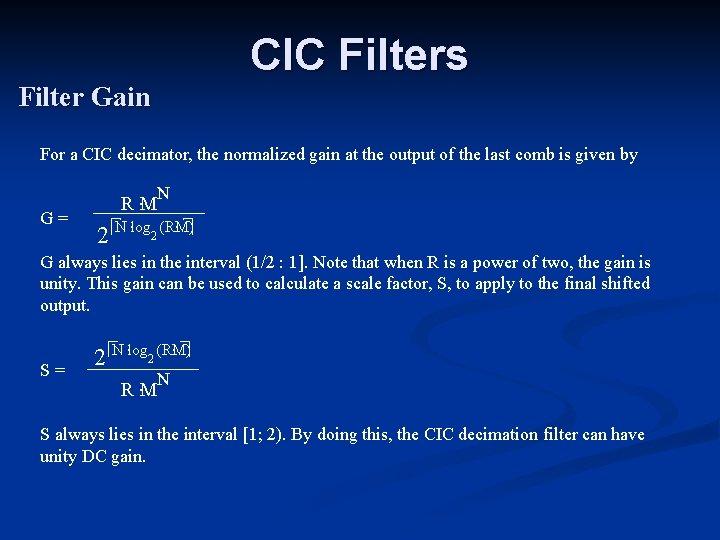CIC Filters Filter Gain For a CIC decimator, the normalized gain at the output of the last comb is given by N G= R. M 2 . N. log (RM) 2 G always lies in the interval (1/2 : 1]. Note that when R is a power of two, the gain is unity. This gain can be used to calculate a scale factor, S, to apply to the final shifted output. S= 2 . N. log (RM) 2 N R. M S always lies in the interval [1; 2). By doing this, the CIC decimation filter can have unity DC gain.CIC Filters Filter Gain For Mercury, the first VARCIC has N = 5 stages, M = 1, and R = 80/160/320 5 G= 5 320 2 5 log 2 320 = 320 242 = . 762939…. G always lies in the interval (1/2 : 1]. Note that when R is a power of two, the gain is unity. This gain can be used to calculate a scale factor, S, to apply to the final shifted output. S= 2 5 log 2 320 5 = 1. 31… 320 S always lies in the interval [1; 2). By scaling this amount, the CIC decimation filter can have unity DC gain.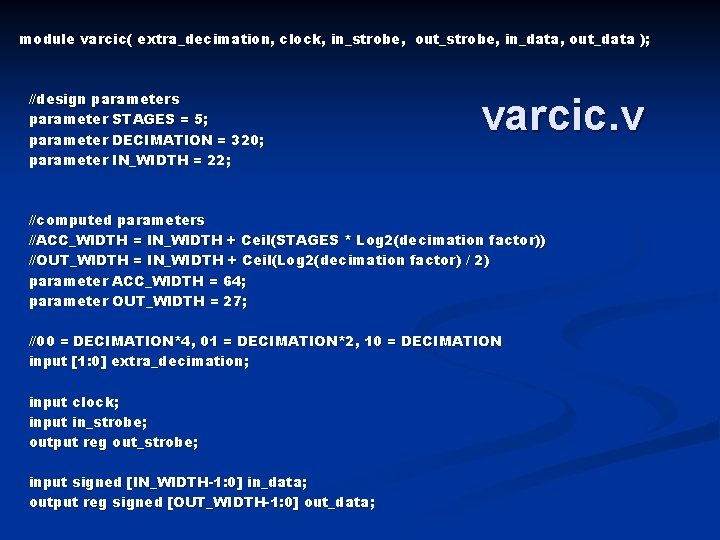module varcic( extra_decimation, clock, in_strobe, out_strobe, in_data, out_data ); //design parameters parameter STAGES = 5; parameter DECIMATION = 320; parameter IN_WIDTH = 22; varcic. v //computed parameters //ACC_WIDTH = IN_WIDTH + Ceil(STAGES * Log 2(decimation factor)) //OUT_WIDTH = IN_WIDTH + Ceil(Log 2(decimation factor) / 2) parameter ACC_WIDTH = 64; parameter OUT_WIDTH = 27; //00 = DECIMATION*4, 01 = DECIMATION*2, 10 = DECIMATION input [1: 0] extra_decimation; input clock; input in_strobe; output reg out_strobe; input signed [IN_WIDTH-1: 0] in_data; output reg signed [OUT_WIDTH-1: 0] out_data;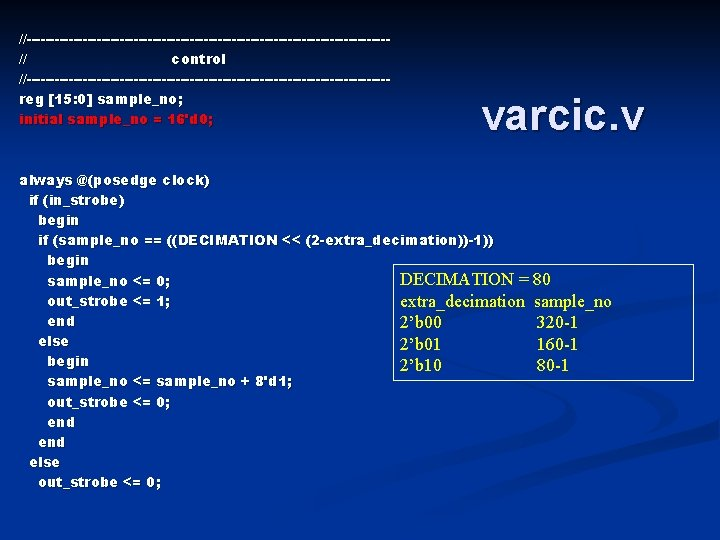//---------------------------------------// control //---------------------------------------reg [15: 0] sample_no; initial sample_no = 16'd 0; varcic. v always @(posedge clock) if (in_strobe) begin if (sample_no == ((DECIMATION << (2 -extra_decimation))-1)) begin DECIMATION = sample_no <= 0; out_strobe <= 1; extra_decimation end 2’b 00 else 2’b 01 begin 2’b 10 sample_no <= sample_no + 8'd 1; out_strobe <= 0; end else out_strobe <= 0; 80 sample_no 320 -1 160 -1 80 -1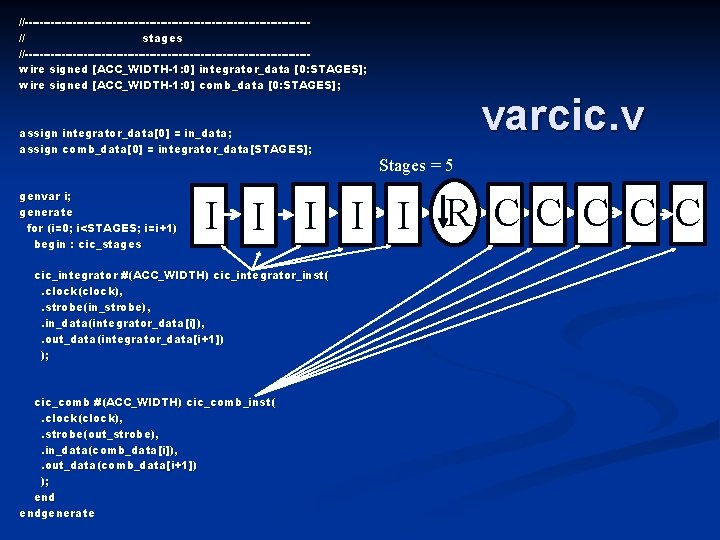//---------------------------------------// stages //---------------------------------------wire signed [ACC_WIDTH-1: 0] integrator_data [0: STAGES]; wire signed [ACC_WIDTH-1: 0] comb_data [0: STAGES]; assign integrator_data = in_data; assign comb_data = integrator_data[STAGES]; genvar i; generate for (i=0; i<STAGES; i=i+1) begin : cic_stages varcic. v Stages = 5 I I I R CC C cic_integrator #(ACC_WIDTH) cic_integrator_inst(. clock(clock), . strobe(in_strobe), . in_data(integrator_data[i]), . out_data(integrator_data[i+1]) ); cic_comb #(ACC_WIDTH) cic_comb_inst(. clock(clock), . strobe(out_strobe), . in_data(comb_data[i]), . out_data(comb_data[i+1]) ); endgenerate//---------------------------------------// output rounding //---------------------------------------localparam MSB 0 = ACC_WIDTH - 1; //63 localparam LSB 0 = ACC_WIDTH - OUT_WIDTH; //41 @ 48 Khz R = 320 Bout = 22 + ceil(5 log 2(320)) Bout = 22 + 42 = 64 OUT_WIDTH = 23 localparam MSB 1 = MSB 0 - STAGES; localparam LSB 1 = LSB 0 - STAGES; //58 //36 localparam MSB 2 = MSB 1 - STAGES; localparam LSB 2 = LSB 1 - STAGES; //53 //31 @ 96 Khz R = 160, Bout = 22 + ceil(5 log 2(160)) Bout = 22 + 37 = 59 @ 192 Khz R = 80 Bout = 22 + ceil(5 log 2(80)) Bout = 22 + 32 = 54 always @(posedge clock) case (extra_decimation) 0: out_data <= comb_data[STAGES][MSB 0: LSB 0] + comb_data[STAGES][LSB 0 -1]; 1: out_data <= comb_data[STAGES][MSB 1: LSB 1] + comb_data[STAGES][LSB 1 -1]; 2: out_data <= comb_data[STAGES][MSB 2: LSB 2] + comb_data[STAGES][LSB 2 -1]; endcase endmodule varcic. vmodule cic_comb( clock, strobe, in_data, out_data ); parameter WIDTH = 64; cic_comb. v input clock; input strobe; input signed [WIDTH-1: 0] in_data; output reg signed [WIDTH-1: 0] out_data; reg signed [WIDTH-1: 0] prev_data; initial prev_data = 0; strobe always @(posedge clock) in_data if (strobe) begin out_data <= in_data - prev_data; prev_data <= in_data; endmodule WIDTH EN prev_data WIDTH + WIDTH EN out_data WIDTH clock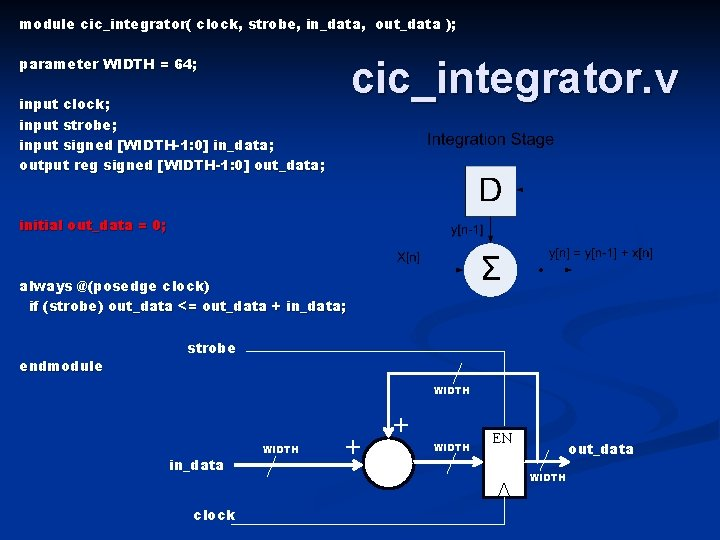module cic_integrator( clock, strobe, in_data, out_data ); cic_integrator. v parameter WIDTH = 64; input clock; input strobe; input signed [WIDTH-1: 0] in_data; output reg signed [WIDTH-1: 0] out_data; initial out_data = 0; always @(posedge clock) if (strobe) out_data <= out_data + in_data; endmodule strobe WIDTH in_data clock WIDTH + + WIDTH EN out_data WIDTH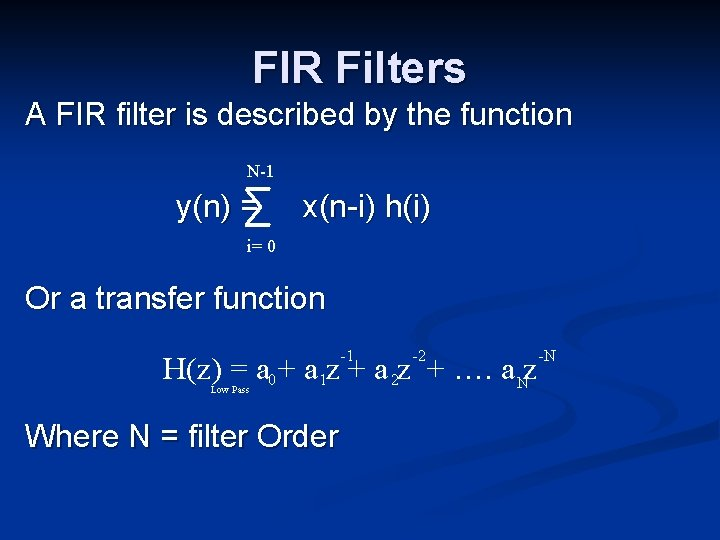FIR Filters A FIR filter is described by the function N-1 y(n) = x(n-i) h(i) i= 0 Or a transfer function -1 -2 H(z) = a 0 + a 1 z + a 2 z + …. a Nz Low Pass Where N = filter Order -NFIR Filters N-1 y(n) = x(n-i) h(i) i = 0 Example: N = 8 x(n) h(0) x(n-7) X h(1) Filter coefficients X h(2) X h(3) X h(4) + y(n) X h(5) X h(6) X h(7) XFIR Filters -1 -2 H(z) = a 0 + a 1 z + a 2 z + …. a Nz -N Low Pass Do how do we calculate the coefficients? For a FIR low pass filter they could be calculated as follows: ai = sin(n. wc ) wc = 2. p. Fc i: 0, 1, …. N n = i – N/2 . w(i) (n. wc ) Fc = cutoff frequency Where w(i) is a window function. Two well known window functions are the Hamming and Blackman Hamming: . . w(i) =. 54 -. 46 cos 2 p n N ( ) Blackman: w(i) =. 42 -. 5 cos 2. p. n N ( ) +. 08 cos 4. p. n N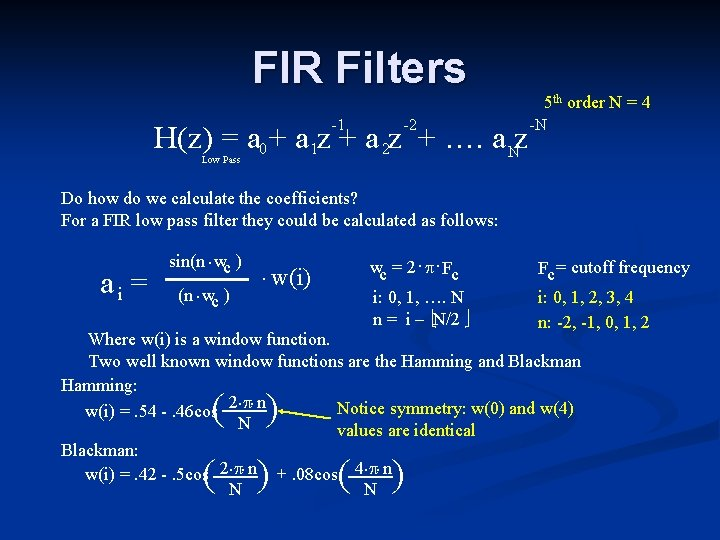FIR Filters -1 -2 H(z) = a 0 + a 1 z + a 2 z + …. a Nz 5 th order N = 4 -N Low Pass Do how do we calculate the coefficients? For a FIR low pass filter they could be calculated as follows: ai = sin(n. wc ) wc = 2. p. Fc i: 0, 1, …. N n = i – N/2 . w(i) Fc = cutoff frequency i: 0, 1, 2, 3, 4 n: -2, -1, 0, 1, 2 Where w(i) is a window function. Two well known window functions are the Hamming and Blackman Hamming: . . Notice symmetry: w(0) and w(4) w(i) =. 54 -. 46 cos 2 p n N values are identical Blackman: w(i) =. 42 -. 5 cos 2. p. n +. 08 cos 4. p. n N N ( ) ( )